# Binary Trees

There is a considerable amount of terminology regarding trees. We will introduce it in the context of the game of Questions (Figure 10-1).

Figure 10-1. The game of Questions. In our implementation, the computer is the guesser.

Questions

Players: 2, the knower and the guesser.

Object: The knower thinks of something and the guesser attempts to determine what it is.

Play: The knower thinks of a person, place, or thing. The guesser asks yes-or-no questions of the knower, who must answer truthfully. Play continues until the guesser wins or gives up.

The variation in which the number of questions is limited (usually to 20) is left as a problem.

In our implementation, several games are played. The program loses at first, but increases its knowledge after each game. A transcript is given in Figure 10-2.

Figure 10-2. Our Questions program gets smarter with every game. We assume that the vast majority of readers have eaten an apple pie, but not a giraffe.

 ``` 1 Welcome to Questions. 2 3 Is it ... a giraffe? no 4 I give up. 5 What is it? a helicopter 6 I need a question to distinguish that from a giraffe. 7 The answer for a helicopter should be yes. 8 Enter the question: Can it fly? 9 Play again (yes or no)? yes 10 11 Can it fly? no 12 Is it ... a giraffe? no 13 I give up. 14 What is it? an apple pie 15 I need a question to distinguish that from a giraffe. 16 The answer for an apple pie should be yes. 17 Enter the question: Have you eaten one? 18 Play again (yes or no)? yes 19 20 Can it fly? no 21 Have you eaten one? yes 22 Is it ... an apple pie? yes 23 I win! ```

After a few hundred games, the program gets pretty good at playing Questions. The program's knowledge is stored in a tree (Figure 10-3).

Figure 10-3. The program's binary decision tree at the end of the session in Figure 10-2.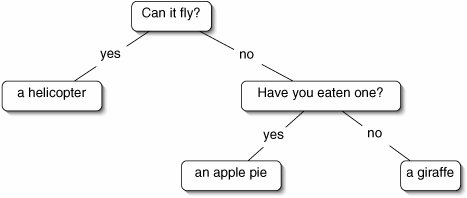Tree Terminology

The tree in Figure 10-3 is a binary tree. Formally, a binary tree is either:

1. empty, or
2. a node with a left subtree and a right subtree. Each of these subtrees is itself a binary tree.

Each rounded rectangle in Figure 10-3 is a node. The entire tree consists of the node labeled "Can it fly?" and two subtrees. The left subtree consists of (the node labeled) "a helicopter," an empty left subtree, and an empty right subtree.

The nodes directly below a node are its children. For example, the two children of "Have you eaten one?" are "an apple pie" and "a giraffe." The most important feature distinguishing binary trees from more general trees (Section 10.3) is that, in a binary tree, no node can have more than two children.

Other family relations follow in the way we would expect. For example, "Can it fly?" is the parent of "a helicopter." Every node in a binary tree has exactly one parent, except for the one at the top, which has no parent. Nodes with the same parent are siblings.

The node at the top of a tree is called the root. While a botanical tree has its root at the bottom, a computer science tree has its root at the top. Nodes with no children are called leaves. Nodes that are not leaves are internal nodes.

The depth of a node is the number of lines (not nodes!) along the path back to the root. Thus, the root is at depth 0, its children are at depth 1, and so on. A level of the tree is the set of nodes at a particular depth. The height of a tree is the depth of the deepest node.

Let's review these terms using the binary tree in Figure 10-4. This tree has height 4, because node J is at depth 4. Node A is the root. The leaves are K, L, F, J, and D. The internal nodes are A, G, H, E, B, I, and C. More information about some of the nodes is given in Figure 10-5.

Figure 10-4. A binary tree with the nodes divided into levels.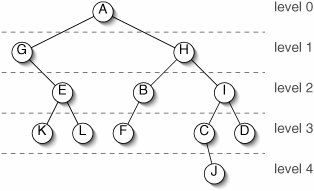Figure 10-5. Information about some of the nodes in Figure 10-4.

Node

Parent

Children

Sibling

Depth

A

G, H

0

B

H

F

I

2

C

I

J

D

3

D

I

C

3

E

G

K, L

2

A node's descendants are itself, its children, their children, and so on. Thus, every node in a tree is a descendant of the root. In Figure 10-4, the descendants of H are H, B, I, F, C, D, and J. A node's proper descendants are all of its descendants except itself.

A node's ancestors are itself, its parent, its grandparent, and so on. In Figure 10-4, the ancestors of L are L, E, G, and A. A node's proper ancestors are all of its ancestors except itself.

The number of nodes in a binary tree depends on the height of the tree and on how "skinny" or "bushy" the tree is (Figure 10-6). At one extreme is a linear tree, where every internal node has only one child. At the other extreme is a perfect binary tree, where all of the leaves are at the same depth and every internal node has exactly two children. (Some texts use the word 'complete' rather than 'perfect.')

Figure 10-6. A linear tree (left) and a perfect binary tree (right). Both of these trees are of height 3.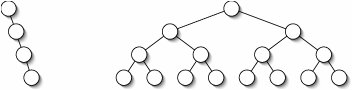The number of nodes in a binary tree of height h can be anywhere between h + 1 (for a linear tree) and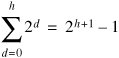(for a perfect binary tree).

Conversely, a binary tree with n nodes has height between log2(n + 1) 1 (for a perfect binary tree) and n 1 (for a linear tree).

These precise formulae are easy to derive from a few examples. The most important thing to remember is that h1. empty, or
2. an item followed by a list.

This definition was implemented in the ListNode class in Section 6.1. Recursively defined structures often lend themselves to linked implementations. We define a class BinaryNode that is similar to ListNode. The difference is that, instead of having a single field next referring to the rest of the list, we have two fields left and right referring to the left and right subtrees.

Since we might have trees of different kinds of things, we again create a generic class. The Questions game needs a tree of Strings. Figure 10-7 shows the linked representation of the decision tree in Figure 10-3.

Figure 10-7. UML instance diagram showing the linked representation of the binary tree in Figure 10-3.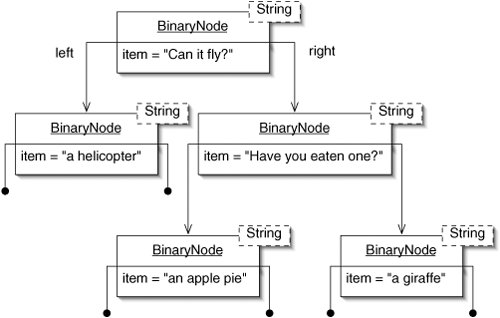The code for the BinaryNode class is given in Figure 10-8.

Figure 10-8. The BinaryNode class. More methods will be added in Section 10.2.

 1 /** Node in a binary tree. */ 2 public class BinaryNode { 3 4 /** The item associated with this node. */ 5 private E item; 6 7 /** The node at the root of the left subtree. */ 8 private BinaryNode left; 9 10 /** The node at the root of the right subtree. */ 11 private BinaryNode right; 12 13 /** Put item in a leaf node. */ 14 public BinaryNode(E item) { 15 this.item = item; 16 // left and right are set to null by default 17 } 18 19 /** Put item in a node with the specified subtrees. */ 20 public BinaryNode(E item, 21 BinaryNode left, 22 BinaryNode right) { 23 this.item = item; 24 this.left = left; 25 this.right = right; 26 } 27 28 /** Return the item associated with this node. */ 29 public E getItem() { 30 return item; 31 } 32 33 /** Return the root of the left subtree. */ 34 public BinaryNode getLeft() { 35 return left; 36 } 37 38 /** Return the root of the right subtree. */ 39 public BinaryNode getRight() { 40 return right; 41 } 42 43 /** Return true if this is a leaf. */ 44 public boolean isLeaf() { 45 return (left == null) && (right == null); 46 } 47 48 /** Replace the item associated with this node. */ 49 public void setItem(E item) { 50 this.item = item; 51 } 52 53 /** Replace the left subtree with the one rooted at left. */ 54 public void setLeft(BinaryNode left) { 55 this.left = left; 56 } 57 58 /** Replace the right subtree with the one rooted at right. */ 59 public void setRight(BinaryNode right) { 60 this.right = right; 61 } 62 63 }

With this structure in hand, we can now write the Questions game. An instance of the Questions class contains a BinaryNode (the root), which may refer in turn to additional BinaryNodes (Figure 10-9).

Figure 10-9. An instance of Questions contains an instance of BinaryNode, which refers to its children, and so on.The easy parts of the program are shown in Figure 10-10.

Figure 10-10. Easy parts of the Questions program.

 ``` 1 import java.util.Scanner; 2 3 /** The game of Questions. */ 4 public class Questions { 5 6 /** For reading from the console. */ 7 public static final Scanner INPUT = new Scanner(System.in); 8 9 /** Root of the decision tree. */ 10 private BinaryNode root; 11 12 /** 13 * Initially, the program guesses that the player is thinking of 14 * a giraffe. 15 */ 16 public Questions() { 17 root = new BinaryNode("a giraffe"); 18 } 19 20 /** Create and repeatedly play the game. */ 21 public static void main(String[] args) { 22 Questions game = new Questions(); 23 System.out.println("Welcome to Questions."); 24 do { 25 System.out.println(); 26 game.play(); 27 System.out.print("Play again (yes or no)? "); 28 } while (INPUT.nextLine().equals("yes")); 29 } 30 31 } ```

Initially, the program's decision tree consists of a single node, containing the String "a giraffe". The tree expands through learningmore on that in the moment. For now, let's imagine that the tree is more elaborate, as in Figure 10-3.

Every internal node in the tree contains a question and every leaf contains a guess as to what the knower has in mind. The play() method starts at the root of the tree, asking the question (or making the guess) stored there. For now, suppose it's a question. If the player answers "yes," the program repeats the process on the left subtree. Otherwise, it goes to the right.

This continues until the program hits a leaf, when it makes a guess. If the guess is correct, the program has won. Otherwise, the program has lost. The play() method is shown in Figure 10-11.

Figure 10-11. The play() method.

 ``` 1 /** Play until the program wins or gives up. */ 2 public void play() { 3 BinaryNode node = root; 4 while (!(node.isLeaf())) { 5 System.out.print(node.getItem() + " "); 6 if (INPUT.nextLine().equals("yes")) { 7 node = node.getLeft(); 8 } else { 9 node = node.getRight(); 10 } 11 } 12 System.out.print("Is it ... " + node.getItem() + "? "); 13 if (INPUT.nextLine().equals("yes")) { 14 System.out.println("I win!"); 15 } else { 16 System.out.println("I give up."); 17 learn(node); 18 } 19 } ```

When the program loses, it learns from experience. This is handled by the learn() method, which replaces all three fields in the leaf node that was an incorrect guess. The field item is replaced by the new question, left is replaced by a new leaf node containing the correct answer, and right is replaced by a new leaf node containing the incorrect guess. No other nodes in the tree are affected. This process is illustrated in Figure 10-12 and the code is in Figure 10-13.

Figure 10-12. A node before (top) and after (bottom) learning. This corresponds to lines 1315 in Figure 10-13.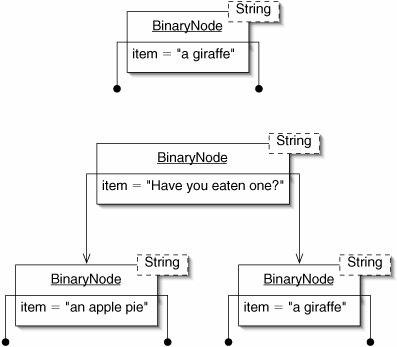Figure 10-13. The learn() method adds two children to a leaf.

 ``` 1 /** 2 * Node is a leaf corresponding to an incorrect guess. Gather 3 * information from the user and add two children to node. 4 */ 5 protected void learn(BinaryNode node) { 6 System.out.print("What is it? "); 7 String correct = INPUT.nextLine(); 8 System.out.println("I need a question to distinguish that from " 9 + node.getItem() + "."); 10 System.out.println("The answer for " + correct 11 + " should be yes."); 12 System.out.print("Enter the question: "); 13 String question = INPUT.nextLine(); 14 node.setLeft(new BinaryNode(correct)); 15 node.setRight(new BinaryNode(node.getItem())); 16 node.setItem(question); 17 } ```

Exercises

 10.1 Is the root of a (nonempty) binary tree always, sometimes, or never a leaf? Explain. 10.2 What are the minimum and maximum number of siblings a node in a binary tree can have? What if the node is the root? 10.3 A ternary tree is just like a binary tree, but each node has three subtrees. What are the maximum and minimum number of nodes in a ternary tree of height h? 10.4 It would be nice to have a method isRoot() for the BinaryNode class which returns true if the node is the root of its tree. Explain why it is not possible to write such a method using the current representation. How might the BinaryNode class be modified to allow such a method to be written? 10.5 Can an internal node in the Questions program's decision tree have only one child? If so, is it the left or right child? If not, why not?

### Tree Traversal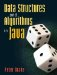Data Structures and Algorithms in Java
ISBN: 0131469142
EAN: 2147483647
Year: 2004
Pages: 216
Authors: Peter Drake

Similar book on Amazon